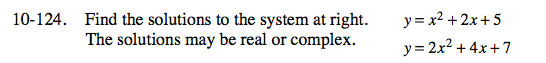### Home > CCA2 > Chapter Ch10 > Lesson 10.2.2 > Problem10-124

10-124.

Find the solutions to the system at right. The solutions may be real or complex. Homework Help ✎ y = x2 + 2x + 5
y = 2x2 + 4x + 7x2 + 2x + 5 = 2x2 + 4x + 7

Set the equation equal to 0 and solve using the Quadratic Formula.

Find the y-value that corresponds to each of your x-values.